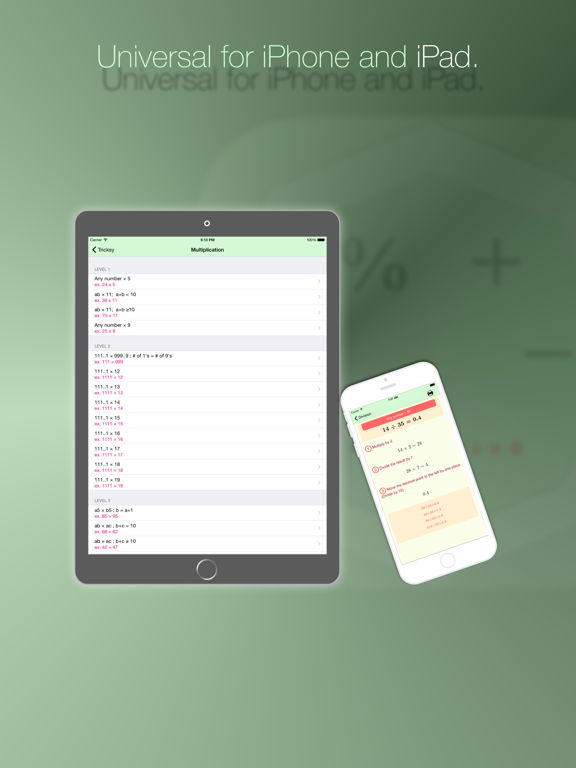クイックアプリ検索 »   新着 | 値下げ | 無料 | 高評価 | 新着オススメ！ | キニナル！ | 新着動画 | iPad新着 | iPad値下げ | iPadオススメ！
APPLICATION INFO - iPhoneアプリの詳細情報
TOP > ゲーム > Tricksy Pro# Tricksy Pro

¥610
Tricksy is a universal app that allows you to solve many math operations in a simple way, by applying untraditional techniques.

This app includes more than 60 lessons classified into these domains: Addition, subtraction, multiplication, division, percentage and power.

These lessons are presented in levels, arranged from the easiest lesson to the most difficult one. Each of these lessons is explained in a simple and clear way; using steps supported by examples.

Besides the lessons, tricksy includes some useful tools for calculation, in which students can benefit, and may apply them during their school domain.

~The included tricks are:

• Adding two-digit numbers like ab + ba
• Adding 2 fractions like 1/a + 1/b
• Adding a sequence of odd numbers beginning by 1: 1 + 3 + 5 + … + 2n-1
• Adding a sequence of even numbers beginning by 2: 2 + 4 + 6 + … + 2n
• Adding a sequence from 1 to any number and back to 1 again: 1 + 2 + 3 +…+ X +…+ 3 + 2 +1
• Adding a sequence of doubles: X + 2⋅X + 2²⋅X + 2³⋅X + ... + 2ⁿ⋅X
• ...

▸ Subtraction:
• Subtracting two-digit numbers like ab − ba
• Subtracting 2 fractions like 1/a − 1/b
• Subtracting 2 fractions like c/a − c/b
• Subtracting 2 fractions like (a − 1)/a − (b − 1)/b
• Subtracting three-digit numbers like abc − cba
• Subtracting the squares of two numbers differing by 2: X² − (X−2)²
• ...

▸ Multiplication:
• Any number × 5
• Two digit number × 11: ab × 11; a + b < 10
• Two digit number × 11: ab × 11; a + b ≥ 10
• Any number × 9
• The repeated #1s × the repeated #9s having the same # of digits: 111..1 × 999..9
• The repeated #1s × 12: 111..1 × 12
• The repeated #1s × 13: 111..1 × 13
• The repeated #1s × 14: 111..1 × 14
• The repeated #1s × 15: 111..1 × 15
• The repeated #1s × 16: 111..1 × 16
• The repeated #1s × 17: 111..1 × 17
• The repeated #1s × 18: 111..1 × 18
• The repeated #1s × 19: 111..1 × 19
• Consecutive decade ending in 5: a5 × b5; b = a + 1
• Two 2-digit numbers with same 10s digit: ab × ac; b + c = 10
• Two 2-digit numbers with same 10s digit: ab × ac; b + c ≠ 10
• Two 2-digit numbers with same 1s digit: ab × cb; a + c = 10
• Two 2-digit numbers with same 1s digit: ab × cb; a + c ≠ 10

▸ Division:
• Any number ÷ 5
• Any number ÷ 50
• Any number ÷ 25
• Any number ÷ 250
• Any number ÷ 125
• Repeating 3-digit number ÷ 37: aaa ÷ 37
• Any number ÷ 40
• Any number ÷ 15
• Any number ÷ 35

▸ Percentage:
• 50% of a number
• 25% of a number
• 20% of a number
• 33.33...% (33⅓%) of a number
• 5% of a number
• 2.5% (2½%) of a number
• 40% of a number
• 60% of a number
• 70% of a number
• 75% of a number
• 45% of a number
• 15% of a number
• ...

▸ Power:
• Number made up of 1s: (111..1)² ; number of digits < 10
• Number made up of 3s: (333..3)²
• Number made up of 6s: (666..6)² ; 1 < number of digits
• Number made up of 9s: (999..9)² ; 1 < number of digits
• Two digit number beginning with 5: (5a)²
• Two digit number ending with 5: (a5)²

Challenge your brain to think efficiently while having fun with our smart math tricks !
--------------------------------------------このアプリはiPhone、iPadの両方に対応しています。
カテゴリー
ゲーム - ファミリー
リリース
2015/1/15

2018/11/9
バージョン
1.4

サイズ
17.9 MB

このバージョンの新機能
• iOS 12 support
•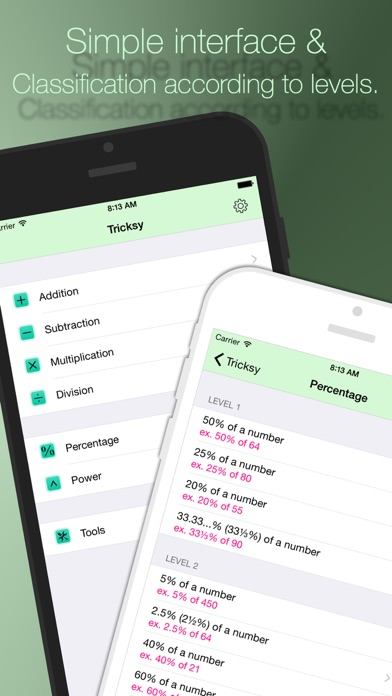•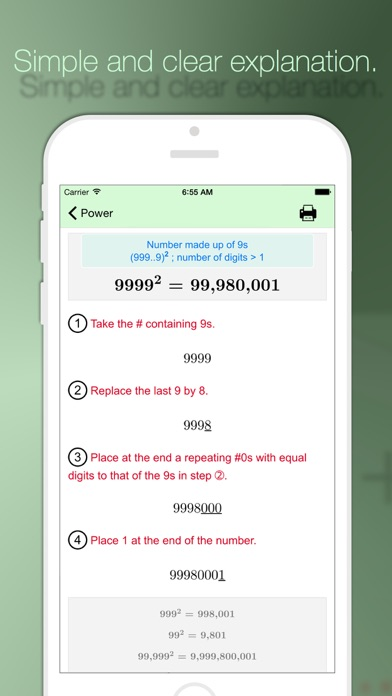•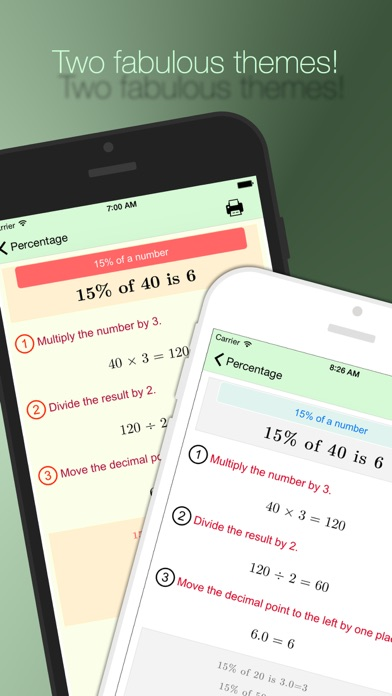•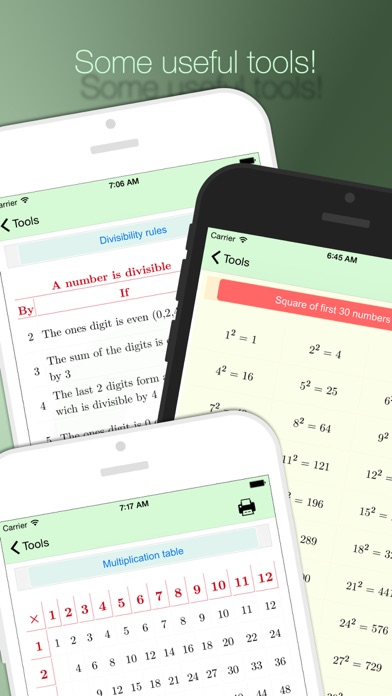•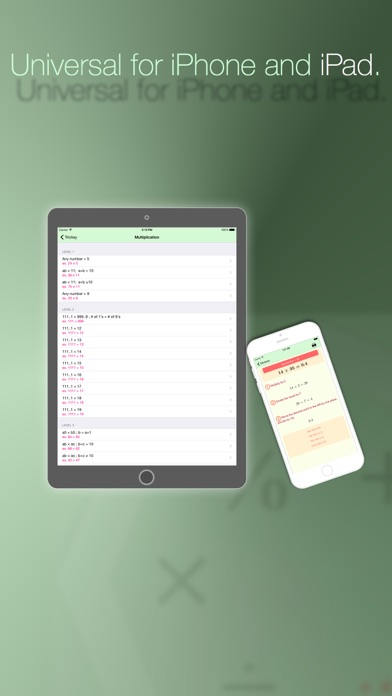•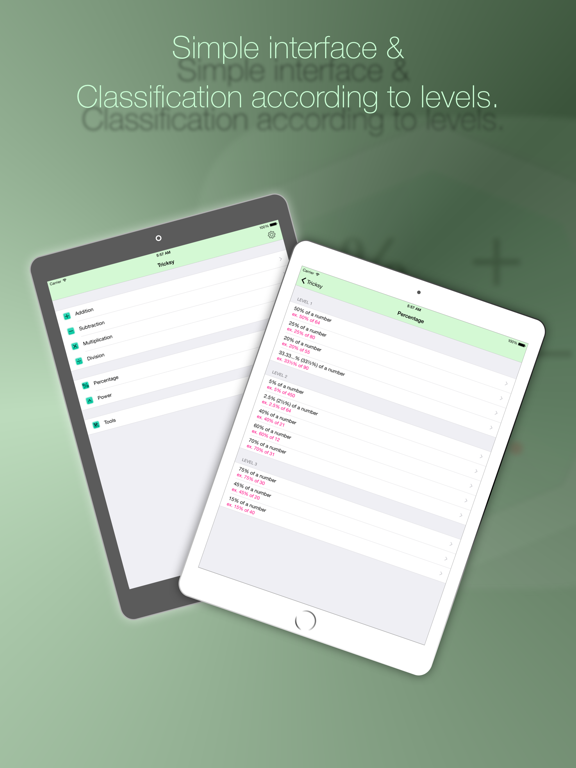•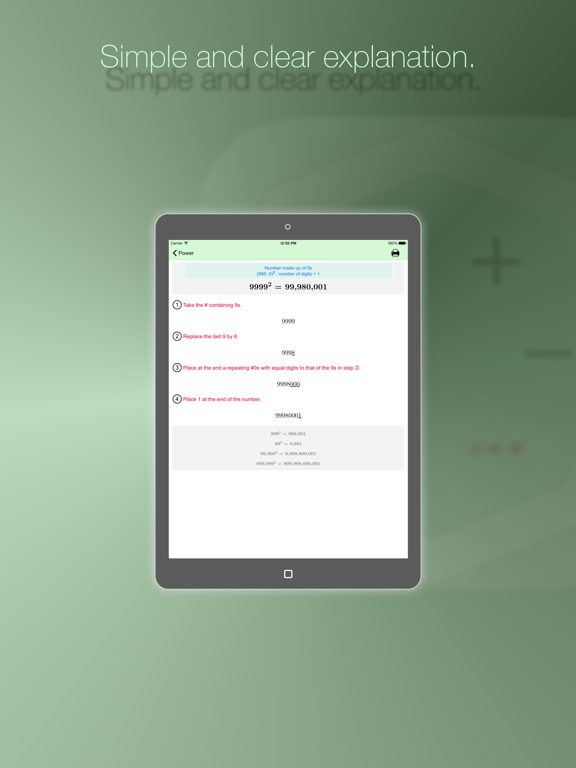•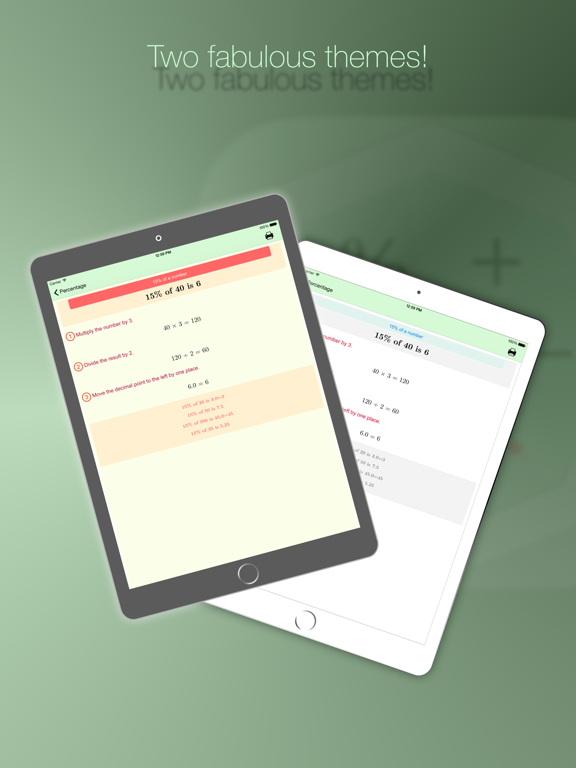•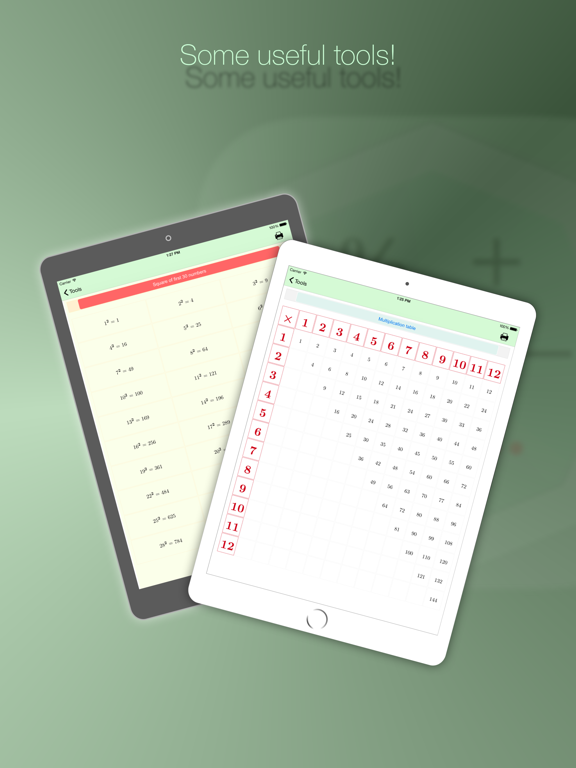•